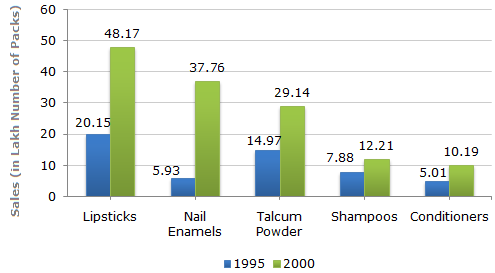# Data Interpretation - Bar Charts - Discussion

A cosmetic company provides five different products. The sales of these five products (in lakh number of packs) during 1995 and 2000 are shown in the following bar graph.

Sales (in lakh number of packs) of five different products of Cosmetic Company during 1995 and 20005.

The sales of conditioners in 1995 was by what percent less than the sales of shampoos in 1995? (rounded off to nearest integer)

 [A]. 57% [B]. 36% [C]. 29% [D]. 25%

Explanation:

Required percentage
 =(7.88 - 5.01) x 100% 7.88
= 36.42%36%.

 Manisha said: (Oct 1, 2014) Why we have divided here with 7.88?

 Ammish said: (Jul 14, 2015) Was wondering same why dividing by 7.88 not 5.01?

 Bulupadhee said: (Jun 9, 2016) Because it has asked for by what percent it is less. So here the formula would be, => ((Big value - Small Value) / Small value) * 100. If this is the case where it was asked by what percent it is greater, the answer might have been, => (7.88 - 5.01 / 5.01) * 100.

 Jain said: (Jun 15, 2019) Because it is written what percent less than shampoo so we took it in the denominator.

 Darsh said: (Aug 21, 2019) Right, Agree @Jain.

 Riva said: (Jul 30, 2021) According to me, the calculation done is wrong, the method and the numbers selected are correct but the correct answer is 57% and not 36%. I have cross-checked and done the calculation more than once. Someone, please verify as well.

 Jigme said: (Aug 16, 2021) 7.88 - 5.01=12.89. 12.89/5.01 * 100= 57.

 Korphu said: (Aug 31, 2021) @Jigme. How come 12.89? Explain.

 Nitin said: (Jan 28, 2022) @Jigme. How, 7.88 - 5.01? Explain this step.